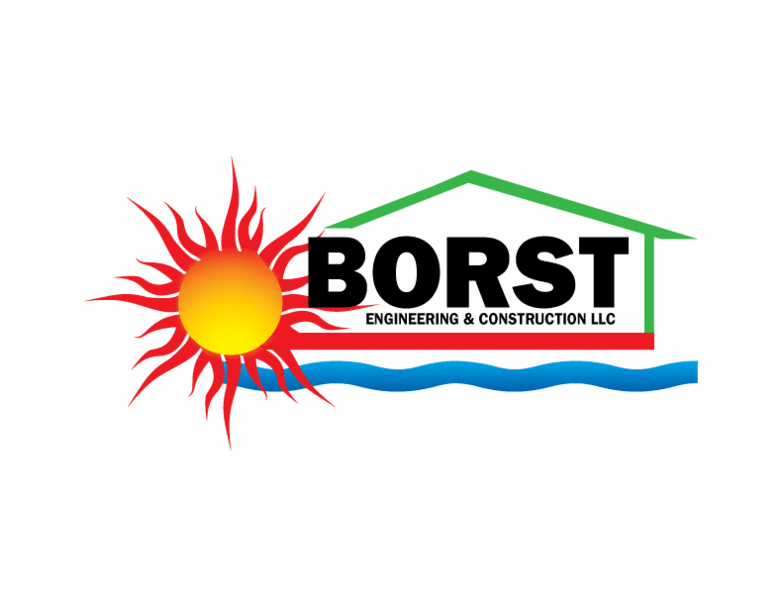Calculator Instructions
Masonry Heater Performance Calculator - Borst Engineering & Construction LLC
 Amount of Wood Burned per Day (Lbs) BTU Value of Wood (BTU/Lb, 6880 for Douglas Fir with 20% moisture content) Heater Overall Efficiency (%, 55-79 typical) Indoor Temp (Degrees F, 68 typical) Initial Facing Temp (Degrees F, 68 typical) Firebox Door Area (SF, 3.5 typical) Facing Area (SF, 100 typical) Facing Thickness (Inches, 5 typical) Facing Density (Lbs/CF, 150 typical) Facing Thermal Conductivity (BTU-Inch/H-SF-Deg F, 14 typical) Facing Specific Heat Capacity (BTU/LB-Deg F, 0.2 is typical) Facing Emissivity (%, 85-95 typical) Facing Convection Heat Transfer Coefficient (BTU/H-SF-Deg F, Still Air is 1.56)
 Total Heat Gain (BTU/Day) Average Heat Gain (BTU/Hour) Facing Volumetric Heat Capacity (BTU/CF-Deg F) Facing Thermal Diffusivity (SF/Hour) Facing Maximum Surface Temp (Degrees F) Facing Maximum Surface Temp Time (Hours after Firing) Heat Gain at 30 Minutes (BTU/Hour) Heat Gain at 1 Hour (BTU/Hour) Heat Gain at 2 Hours (BTU/Hour) Heat Gain at 4 Hours (BTU/Hour) Heat Gain at 6 Hours (BTU/Hour) Heat Gain at 8 Hours (BTU/Hour) Heat Gain at 10 Hours (BTU/Hour) Heat Gain at 12 Hours (BTU/Hour) Heat Gain at 14 Hours (BTU/Hour) Heat Gain at 16 Hours (BTU/Hour) Heat Gain at 18 Hours (BTU/Hour) Heat Gain at 20 Hours (BTU/Hour) Heat Gain at 22 Hours (BTU/Hour) Heat Gain at 24 Hours (BTU/Hour)
Borst Engineering & Construction LLC